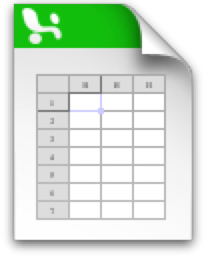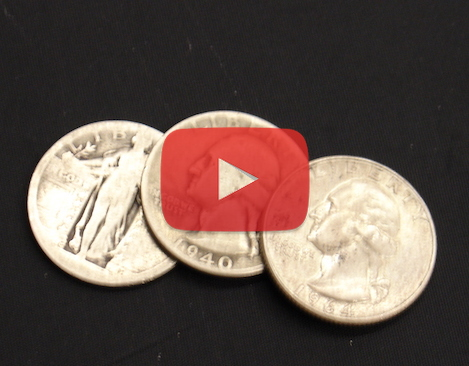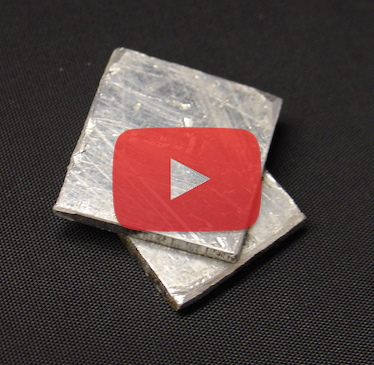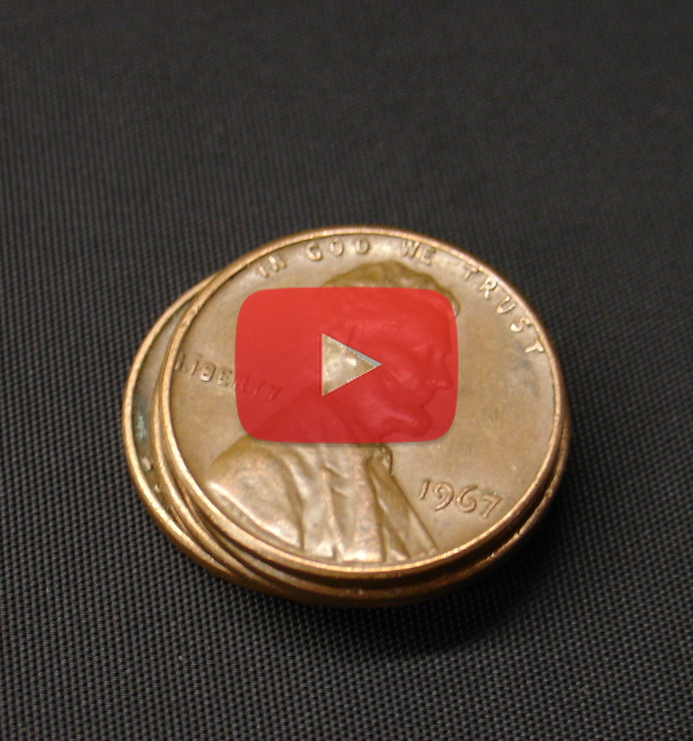# Radioactivity Virtual LaboratoryNeutron Activation and Decay Experiments

1. ## Laboratory Manual and Instructions

1. ### Introduction

Welcome to this Website that contains experiments on radioactivity, neutron activation and radioactive decay. The primary purpose of this site is to provide a resource about the neutron activation process and how it can be used to create and identify radioactive isotopes. These videos and accompanying data contain a range of introductory radioactive experiments for students. These experiments offer a unique opportunity for students in institutions such as high schools or small colleges where a neutron source would be impractical or impossible to maintain. In the event that others will find this material useful, this manual has been created to act as a guide for possible pre-lab learning, laboratory experiments, homework activities, and demonstrations. The random access nature of this page and streaming video allow teachers to easily show only those parts of the video that will work best in their course or laboratory as well as contain all relevant data, images, and documentation. The experiments described on this page and accompanying videos below were filmed in 2005 and turned into a DVD and then converted into this on-line resource in 2016.

These videos were initially created to address the imminent removal of a PuBe "Neutron Howitzer" source that had been at the University of Wisconsin - Whitewater since 1968. With the impending loss of this source, students would be unable to perform a standard introductory laboratory activity, namely measurements of the radioactive decay of activated silver quarters. In this experiment, silver US quarters (c. 1940) were irradiated with neutrons, causing the stable silver atoms to turn into unstable silver atoms. Students then used a Geiger counter apparatus to repeatedly measure the decay counts occurring during a certain length of time, then plotted the decay rate over time and determined the half-life of the unstable silver atom. Although this activity had been an instructive and popular laboratory exercise, maintaining a 5 Curie source for the sole purpose of this one laboratory had become too burdensome to justify continued possession (and payment of additional license fees.)

A video recording of this popular laboratory was made so that students would still be able to view the laboratory. Recording the neutron activation process and using computer data acquisition during the experiment shows situations that were logistically not possible in our ordinary laboratory activity. Recording a set of other appropriate elements, some of which have a longer half-life was also conducted. In this manner, a virtual laboratory set has been created. In a single standard laboratory session, or as a homework activity, different student groups can watch different, but similar, experiments, analyze the recorded data from the different elements, and determine the half-life for their assigned element. In addition, several different sources show different shielding characteristics for α, β, and γ emissions.

2. ### Laboratory Activities and Worksheets.

1. Background Information about Nuclear Decay

These activities are intended for students in a general or conceptual physic course.
 Introduction Activity:Understanding Half-Life Activity (.pdf)Understanding Half-Life Excel file (.xlsx)Understanding Half-Life Teachers note (.pdf) Here is an introductory item that can be given to students to work on in class before the laboratory experiment. The "Understanding Half-life" activity provides a short experiment to act as a guide for basic understanding of half-life.Summary information: Atoms, Radioactive Decay, and Neutron Activation.(.pdf) This one-page handout provides summary information about atoms, the radioactive decay process, notation on writing elements, and the neutron activation process.A worksheet for students watching the introductory videos. (.pdf) This worksheet can be given to students as an activity to maintain attention and reinforce their understanding and comprehension of the material presented in the About atoms, radiation, and half-life and The Neutron Activation Process slide shows. Students can complete this worksheet while watching the slides.
2. General Physics Course Activity

The following activity was designed for students in a one-semester survey of physics course. It uses the concepts of averages, natural logarithms, and exponentials.Introductory Tutorial relating to nuclear reactions (.pdf) This is a small-group activity relating to radioactive decay. This activity takes approximately 60 minutes for groups to complete depending on their level. Solutions to the tutorial are provided.
3. Laboratory Instructions40 min. Lab Instructions (.pdf) This is an introductory physics level activity that can be accomplished in 40 minutes relating to the experiments on this Website. The students watch a video relating to their chosen element and complete the laboratory worksheet to determine the element's half-life. Students will need access to the Student_Decay_Data_Simple.xls or Student_Decay_Data.xls data file.2 hr. Lab (Algebra-based) This laboratory is suitable for students in a two or three-hour laboratory session for a two-semester algebra based physics course. This laboratory activity involves more analysis than the above lab. Students will need access to the Student_Decay_Data_Simple.xls or Student_Decay_Data.xls3 hr. Lab (Calculus-based) This is a general guideline for students in a calculus-based physics course. This activity assumes more independent and student-directed work than the those above. It is designed for Introductory Calculus-based Physics / Advanced Activities with a three-hour lab session. Students will need access to the Student_Decay_Data_Simple.xls or Student_Decay_Data.xls

3.

4. ## Neutron Activation and Decay Experiments

5. ### Downloadable Excel (.xls and .xlsx) tables containing all of the data shown in the videos.

There are two data files to choose from. The first contains a simplified data analysis area appropriate for introductory (algebra-based physics) students. The second has the same data, but the analysis area allows for more sophisticated calculations as would be appropriate for a calculus-based course or independent study.

### Videos of element neutron activation and recording of decay experiment.

Watch the videos below to observe how objects can become radioactive through neutron activation and to observe the rate of decay over time. Data from each experiment can be downloaded separately as .xls tables below, or use the data tables above for the complete set.

ElementData shown in video

### Silver (Ag)Video of Ag ExperimentAg Decay Data (.xlsx)

### Aluminium (Al)Video of Al ExperimentAluminum Decay Data (.xlsx)

### Copper (Cu)Video of Cu ExperimentCopper Decay Data (.xlsx)

### Indium (In)Video of In ExperimentIn Decay Data (.xlsx)

### New Pennies

(unknown element)Video of Pennies ExperimentPennies Decay Data (.xlsx)